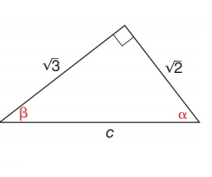Chapter 11.2, Problem 5E### Elementary Geometry for College St...

6th Edition
Daniel C. Alexander + 1 other
ISBN: 9781285195698

#### Solutions

Chapter
Section### Elementary Geometry for College St...

6th Edition
Daniel C. Alexander + 1 other
ISBN: 9781285195698
Textbook Problem
1 views

# In Exercises 1 to 6, find cos α and cos β .To determine

To find:

The cosα and cosβ.

Explanation

Consider the following figure,

General formula for cosine ratio is given below,

From the given figure the value c is the hypotenuse value since it is opposite to right angle.

Then 2 is the length of the leg adjacent to α and 3 is the length of leg adjacent to β.

The Pythagorean theorem is given below,

(hypotenuse)2=(lengthofoneleg)2+(lengthofotherleg)2

Substitute the values of hypotenuse=c,lengthofoneleg=3 andlengthofotherleg=2 to get the following,

### Still sussing out bartleby?

Check out a sample textbook solution.

See a sample solution

#### The Solution to Your Study Problems

Bartleby provides explanations to thousands of textbook problems written by our experts, many with advanced degrees!

Get Started

#### Find more solutions based on key concepts